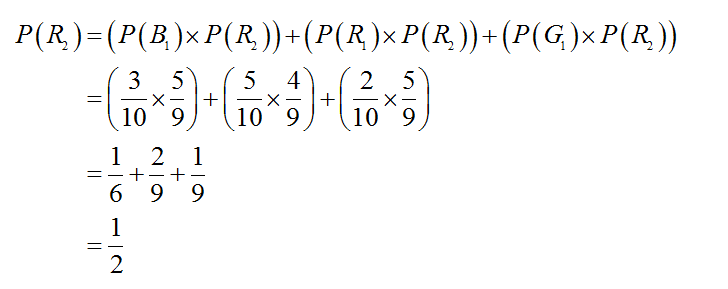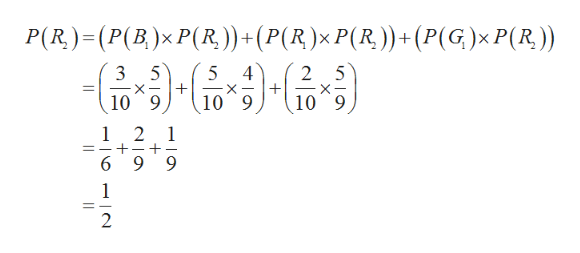# Assume that the box contains 10 balls: 5 red, 2 green, and 3 blue. Two balls are drawn at random, one after the other without replacement.What is the probability that the first ball was blue, given that the second was red?

Question

Assume that the box contains 10 balls: 5 red, 2 green, and 3 blue. Two balls are drawn at random, one after the other without replacement.

What is the probability that the first ball was blue, given that the second was red?

check_circle

Step 1

The probability that first was blue and given that second was red is,

Subscripts 1 and 2 represent first draw and second draw.

Step 2

Now, the value of P(R2) is,...help_outlineImage TranscriptioncloseP(R)-(P(B)x P(R)) + (P(R)x P(R)) + (P (G)x P(R.)) 5 5 4 2 5 X X 10 9 10 9 10 9 1 2 1 _ 6 9 9 1 2 fullscreen

### Want to see the full answer?

See Solution

#### Want to see this answer and more?

Solutions are written by subject experts who are available 24/7. Questions are typically answered within 1 hour.*

See Solution
*Response times may vary by subject and question.
Tagged in

### Math